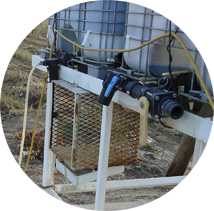# Chemigation Calculators

Chemical Injection Rate (Volume): Calculate the injection rate based on the volume per area.

Chemical Injection Rate (Mass): Calculate the injection rate based on the weight per area.

Chemical Injection Rate (Water Chemistry Control): Calculate the the injection rate for water chemical solutions.

Maximum Solution Concentration: Find the solution concentration in the irrigation lines for a given chemical injection rate and water flow rate.

Mixing Dry Chemicals: Calculate how much dry chemical to mix with water, depending on your water requirements.

Batch/Bulk Application: Calculates the chemical injection rate for batch or bulk chemical applications.

Minimum Batch Volume: Minimum amount of water that must be mixed with dry chemicals in order to get the chemicals dissolved into solution.

Calibration: Calibrate your injection pumps by calculating the estimated fill time of a container using the pump's injection rate.

Quantity Per Sprinkler Setting: Determine the irrigated area, and either the fertilizer or the herbicide application based on the characteristics of the area's irrigation system.WSU Prosser - IAREC, 24106 N Bunn Rd, Prosser WA 99350-8694, 509-786-2226,